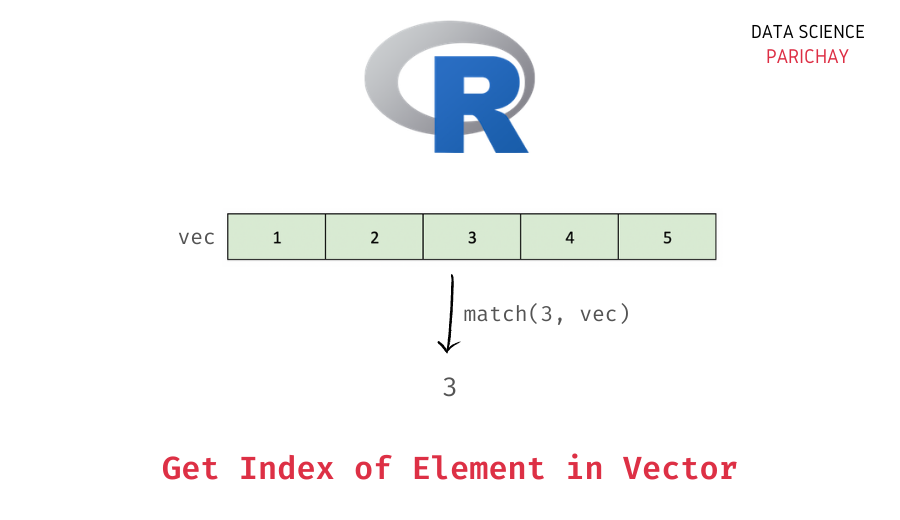# R – Get Index of an Element in a Vector

In this tutorial, we will look at how to get the index of an element in an R vector with the help of some examples.

## How to get the index of an element in an R vector?

You can use the `match()` function in R, to get the index of an element in a vector. It returns the index of the first occurrence of the value in the vector as an integer. The following is the syntax –

```# get index of element e in vector vec
match(e, vec)```

Pass the element (or the vector) as the first argument and the vector in which you want the know the position as the second argument.

You can also use the `match()` function to get the index of multiple values at a time in a vector (see the examples below).

## Examples

Let’s look at some examples of getting the index (or position) of an element in an R vector. First, we will create a vector that we will be using throughout this tutorial.

```# create a vector
vec <- c("a", "b", "c", "d", "c")
# display the vector
print(vec)```

Output:

` "a" "b" "c" "d" "c"`

We have a vector of some characters.

### Index of the first occurrence of an element in a vector

Let’s use the `match()` function to get the position of the “c” in the above vector. For this, pass “c” as the first argument, and the vector, vec as the second argument to the function.

📚 Data Science Programs By Skill Level

Introductory

Intermediate ⭐⭐⭐

🔎 Find Data Science Programs 👨‍💻 111,889 already enrolled

Disclaimer: Data Science Parichay is reader supported. When you purchase a course through a link on this site, we may earn a small commission at no additional cost to you. Earned commissions help support this website and its team of writers.

```# get index of "c"
match("c", vec)```

Output:

`3`

You can see that we get the position (or index) of “c” as 3. Note that in the above vector, “c” occurs at positions 3 and 5 but we only get the index of its first occurrence (or match) with the `match()` function.

If you want to get all the indexes of occurrences of an element in a vector R, use the `which()` function instead. Pass an equality operation (checking whether the vector value is the same as the element we want the indexes for) as the argument.

```# get all indexes of "c"
which(vec == "c")```

Output:

`3 5`

You can see that we get all the positions for the element “c” in the above vector.

### Index of the first occurrence of multiple elements in a vector

You can also pass a vector as the first argument to the `match()` function. In such a case, the match function will get the positions of the first occurrence of each element in that vector.

For example, let’s get the positions of “a” and “c” in the above vector using the `match()` function.

```# get index of "a" and "c"
match(c("a", "c"), vec)```

Output:

`1 3`

We the position of “a” as 1 and “c” as 3.

You might also be interested in –

•